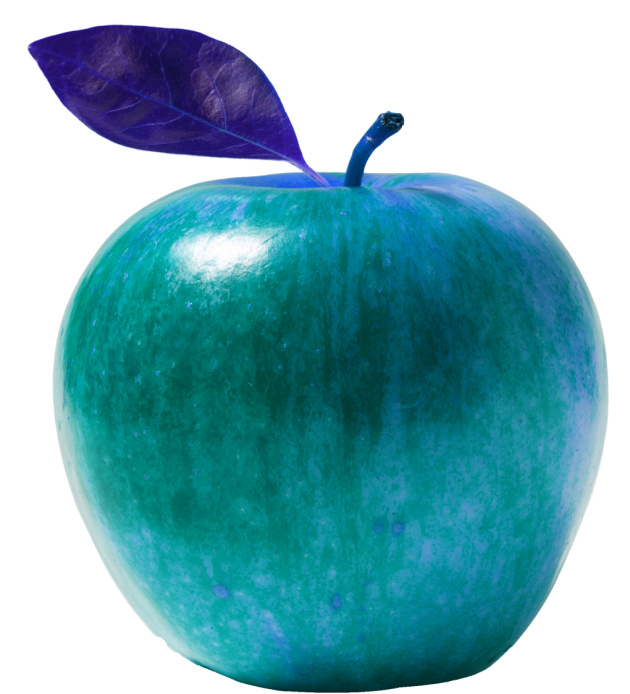---

# INVENTIONSSingle Digit Glyphs are a way to express any possible number (no matter how big) by using a single symbol.Example: number 137 expressed with a Single Digit Glyph

Any number can be expressed with a Single Digit Glyph through the following steps:
1) Convert the number to its binary form.
2) Check the first digit: if it's 0, draw a straight horizontal line going from the bottom left to the bottom right of an empty cell; if it's 1, draw a straight vertical line going from the bottom left to the top left of the cell.
3) For each subsequent digit, draw a straight line perpendicular to the last one; if the digit is a 0, the line starts from the ending point of the last one, if the digit is a 1, the line starts near the starting point of the last one; in both cases, the line must not touch any line other than the last one.
4) The dot between unit digits and tenth digits is represented by a bold dot at the point where the corresponding lines meet.How to convert a number into its Single Digit GlyphExamples: natural numbers from 1 to 100 expressed with Single Digit GlyphsFirst published: 08/01/2020.

Last edit: 09/01/2020.